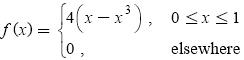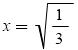# Finding the mode of a distribution with calculus.

The probability density function of the random variable X is defined byWhat is the probability that three independent observations from the distribution of X are all less than the mode of X?

This is a question I got incorrect. I have very little experience with mode and I understand that it's the value of the random variable with the highest probability. The solution for finding the mode is as follows:

The max point occurs when f '(x) = 0This is the max point or mode because f ''(x) is a negative number.

Can somebody explain to me their solution in more detail please? I understand that when the first derivative is zero, the curve is either at the maximum or minimum. But how would I tell which one? Also, how do I know it's the absolute maximum?

thank you,

• You just found the x-coordinate of the maximum point. Plug this value into f(x) to find the y-coordinate of the maximum point Jul 14, 2017 at 18:30
• You also have to make sure that $f''(\sqrt{\frac{1}{3}})\le 0$ in this case to conclude that $x=\sqrt{\frac{1}{3}}$ is the 'max point'. Jul 14, 2017 at 18:31
• Thank you @StubbornAtom Jul 14, 2017 at 18:33
• @GamrCorps For this application we don't really need to know the y-coordinate at the maximum of $f(x).$ We just need to know the range of $x$ values over which to integrate the distribution, which in this problem starts at $0$ and ends at $1/\sqrt3.$ Jul 14, 2017 at 18:33

You're correct that the first derivative being 0 means that you have an extremum (maximum or minimum). The solution then mentions that f"(x) is a negative number, which makes it a maximum.

Remember that f"(x) describes the curvature of the function. If it is positive, the function is curving "up, like a cup" and if it is negative, the function is curving "down, like a frown." You should be able to visualize which of these would result in a local maximum.

How, then, do you know it is a global maximum? The nice thing about continuous functions on closed intervals (here the interval is [0,1]) is that they will achieve their maximum value EITHER at the endpoints of the intervals, or at a local extremum, but nowhere else. So to be absolutely certain, you could check the value of the function at the endpoints as well and compare.

• I appreciate your comprehensive answer. thank you Jul 14, 2017 at 18:36
• Technically, an arbitrary function $f$ could also have an inflection point at which $f'$ could be zero without being either a local minimum or a local maximum. (For example, consider $y=x^3$ at $x=0.$) The negative value of $f''$ rules this out, however. Jul 14, 2017 at 18:36

The global maximum of a continuous and differentiable real-valued function $f(x)$ on some interval $[a,b]$ occurs either at one of the endpoints, or at a critical point $x = x_0$ satisfying $f'(x_0) = 0$. Thus, by computing the derivative and finding the set of critical points, you obtain a set of candidate extrema, after which you can use other arguments to find which one(s) correspond to a global maximum of $f$.

So in this case, you know that you are restricted to $[a,b] = [0,1]$. You also know that $f(x) \ge 0$ on this interval. You can compute fairly trivially that $f(0) = f(1) = 0$. After finding that $x_0 = 1/\sqrt{3}$ gives $f'(x_0) = f'(1/\sqrt{3}) = 0$, and $f(1/\sqrt{3}) > 0$, you know that this is a critical point and it is better than the endpoints, so the global maximum is not at either endpoint. And since it is the only critical point on the given interval, it certainly isn't a minimum, so it's a maximum.

To be even more sure, you can calculate the second derivative $f''(x) = -24x$, and you can conclude that on $x \in [0,1]$, this function is always nonpositive, thus the function is concave down. Thus the mode is $X = 1/\sqrt{3}$ as claimed. The probability that a single observation is less than the mode is $$\Pr[X < 1/\sqrt{3}] = \int_{x=0}^{1/\sqrt{3}} f_X(x) \, dx = \int_{x=0}^{1/\sqrt{3}} 4(x-x^3) \, dx.$$ The probability that three independent observations are all each less than the mode is simply $$\Pr\left[\bigcap_{i=1}^3 X_i < \frac{1}{\sqrt{3}} \right] \overset{\text{iid}}{=} \Pr[X < 1/\sqrt{3}]^3,$$ that is to say, it is simply the probability of a single observation being less than the mode, raised to the third power.